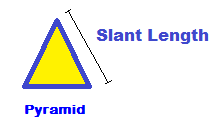## Surface Area of a Square Pyramid Calculator

Find the Surface Area of a Square Pyramid with this calculator.

Base Width:
Height:
Fill in the width of the square base of a regular pyramid, and the
height of the regular pyramid to find the surface area.

## How do you find the surface area of a pyramid with a square base?

To find the surface area, use this square pyramid surface area formula:
Square Pyramid Surface Area = 2 x B x S + B2

B = Width of the Square Base

S = Slant length of one of the triangular faces and is calculated from
the height and base width by using the equation:

S= The square root of [(.5B)2 + Height2]Example: The surface area of a regular square pyramid with base width
of 6 inches, and height of 8 inches:

S= square root of (9 +64)

S= 8.544

Surface Area = 2 x 6 x 8.544 + 36

Calculated out this gives a surface area of 138.528 Square Inches.